# HSSlive: Plus One & Plus Two Notes & Solutions for Kerala State Board

## BSEB Class 9 Maths Chapter 7 Triangles Ex 7.3 Textbook Solutions PDF: Download Bihar Board STD 9th Maths Chapter 7 Triangles Ex 7.3 Book AnswersBSEB Class 9 Maths Chapter 7 Triangles Ex 7.3 Textbook Solutions PDF: Download Bihar Board STD 9th Maths Chapter 7 Triangles Ex 7.3 Book Answers

BSEB Class 9th Maths Chapter 7 Triangles Ex 7.3 Textbooks Solutions and answers for students are now available in pdf format. Bihar Board Class 9th Maths Chapter 7 Triangles Ex 7.3 Book answers and solutions are one of the most important study materials for any student. The Bihar Board Class 9th Maths Chapter 7 Triangles Ex 7.3 books are published by the Bihar Board Publishers. These Bihar Board Class 9th Maths Chapter 7 Triangles Ex 7.3 textbooks are prepared by a group of expert faculty members. Students can download these BSEB STD 9th Maths Chapter 7 Triangles Ex 7.3 book solutions pdf online from this page.

## Bihar Board Class 9th Maths Chapter 7 Triangles Ex 7.3 Books Solutions

 Board BSEB Materials Textbook Solutions/Guide Format DOC/PDF Class 9th Subject Maths Chapter 7 Triangles Ex 7.3 Chapters All Provider Hsslive

## How to download Bihar Board Class 9th Maths Chapter 7 Triangles Ex 7.3 Textbook Solutions Answers PDF Online?

2. Click on the Bihar Board Class 9th Maths Chapter 7 Triangles Ex 7.3 Answers.
3. Look for your Bihar Board STD 9th Maths Chapter 7 Triangles Ex 7.3 Textbooks PDF.
4. Now download or read the Bihar Board Class 9th Maths Chapter 7 Triangles Ex 7.3 Textbook Solutions for PDF Free.

Find below the list of all BSEB Class 9th Maths Chapter 7 Triangles Ex 7.3 Textbook Solutions for PDF’s for you to download and prepare for the upcoming exams:

## BSEB Bihar Board Class 9th Maths Solutions Chapter 7 Triangles Ex 7.3

Question 1.
∆ ABC and ∆ DBC are two isosceles triangles on the same base BC and vertices A and D are on the same side of BC (see figure). If AD is extended to intersect BC at P, show that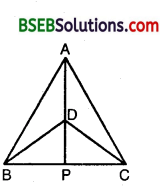(i) ∆ ABD ≅ ∆ ACD
(ii) ∆ ABP ≅ ∆ ACP
(iii) AP bisects ∠A as well as ∠D.
(iv) AP is the perpendicular bisector of BC.
Solution:
(i) In ∆s ABD and DBC, we have
AB = AC [Given]
BD = DC [Given]
∴ By SSS criterion of congruence, we have
∆ ABD ≅ ∆ ACD.

(ii) In ∆s ABP and ACP, we have
AB = AC [Given]
∠BAP = ∠PAC
[∵ ∆ ABD = ∆ ACD ⇒ ∠B AD = ∠DAC ⇒ ∠BAP = ∠PAC]
and, AP = AP [Common]
∴ By SAS criterion 6f congruence, we have
∆ ABP ≅ ∆ ACP.

(iii) Since ∆ ABD = ∆ ACD. Therefore,
⇒ AD bisects ∠A ⇒ AP bisects ∠A … (1)
In ∆s BDP and CDP, we have
BD = CD [Given]
BP = PC [∵ ∆ ASP = ∆ ACP ⇒ BP = PC]
and DP = DP [Common]
∴ By SSS criterion of congruence, we have
∆ BDP ≅ ∆ CDP
⇒ ∠BDP = ∠PDC
⇒ DP bisects ∠D ⇒ AP bisects ∠D … (2)
Combining (1) and (2), we get
AP bisects ∠A as well as ∠D.

(iv) Since AP stands on BC
∴ ∠APB + ∠APC = 180° [Linear Pair]
But ∠APB = ∠APC [Proved above]
∴ ∠APB = ∠APC = 180°2 = 90°
Also , BP = PC [Proved above]
⇒ AP is perpendicular bisector of BC.

Question 2.
AD is an altitude of an isosceles triangle ABC in which AB = AC. Show that (i) AD bisects BC, (ii) AD bisects ∠A.
Solution:
AD is the altitude drawn from vertex A of an isosceles ∆ ABC to the opposite base BC so that AB = AC, ∠ADC = ∠ADB = 90°.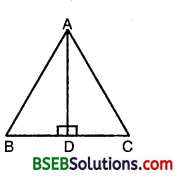Hyp. AB = Hyp. AC [Given]
∴ By RHS criterion of congruence, we have
⇒ BD = DC and ∠BAC = ∠DAC
[∵ Corresponding parts of congruent triangles are equal]
Hence, AD bisects BC, which proves (i), and AD bisects ∠A, which proves (ii).

Question 3.
Two sides AB and BC and median AM of one triangle ABC are respectively equal to sides PQ and QR and median PN of ∆ PQR (see figure). Show that:
(i) ∆ ABM ≅ ∆ PQN
(ii) ∆ ABC ≅ ∆ PQR.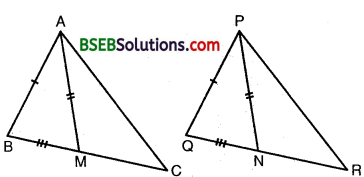Solution:
Two ∆s ABC and PQR in which AB = PQ, BC = QR and AM = PN.
Since AM and PN are medians of As ABC and PQR respectively.
Now, BC = QR [Given]
⇒ 12BC = 12QR ⇒ BM = QN … (1)
Now, in ∆s ABM and PQN, we have
AB = PQ [Given]
BM = QN [From (i)]
and, AM = PN [Given]
∴ By SSS criterion of congruence, we have ∆ ABM = ∆ PQN, which proves (i)
⇒ ∠B = ∠Q … (2)
[∵ Corresponding parts of congruent triangles are equal]
Now, in ∆s ABC and PQR, we have
AB = PQ [Given]
∠B = ∠Q [From (2)]
BC = QR [Given]
∴ By SAS criterion of congruence, we have
∆ ABC = ∆ PQR, which proves (ii).

Question 4.
BE and CF are two equal altitudes of a triangle ABC. Using RHS congruence rule, prove that the triangle ABC is isosceles.
Solution:
In ∆s BCF and CBE, we have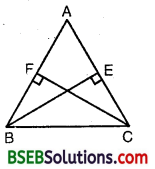∠BFC = ∠CEB [∵ Each = 90°]
Hyp. BC = Hyp. BC [Common]
FC = EB
∴ By R.H.S. criterion of congruence, we have
∆ BCF ≅ ∆ CBE
⇒ ∠FBC = ∠ECB
[∵ Corresponding parts of congruent triangles are equal]
Now, in ∆ ABC
∠ABC = ∠ACB [∵∠FBC = ∠ECB]
⇒ AB = AC [∵ Sides opposite to equal angles of a triangle are equal]
∴ ∆ ABC is an isosceles triangle.

Question 5.
ABC is an isosceles triangle with AB = AC. Draw AP ⊥ BC to show that ∠B = ∠C.
Solution:
In ∆s ABP and ACP, we have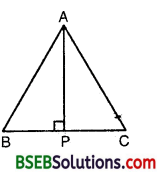AB = AC [Given]
AP = AP [Common]
and ∠APB = ∠APC [∵ Each = 90°]
∴ By R.H.S. criterion of congruence, we have
∆ ABP ≅ ∆ ACP
⇒ ∠B = ∠C [∵ Corresponding parts of congruent triangles are equal]

## Bihar Board Class 9th Maths Chapter 7 Triangles Ex 7.3 Textbooks for Exam Preparations

Bihar Board Class 9th Maths Chapter 7 Triangles Ex 7.3 Textbook Solutions can be of great help in your Bihar Board Class 9th Maths Chapter 7 Triangles Ex 7.3 exam preparation. The BSEB STD 9th Maths Chapter 7 Triangles Ex 7.3 Textbooks study material, used with the English medium textbooks, can help you complete the entire Class 9th Maths Chapter 7 Triangles Ex 7.3 Books State Board syllabus with maximum efficiency.

## FAQs Regarding Bihar Board Class 9th Maths Chapter 7 Triangles Ex 7.3 Textbook Solutions

#### Can we get a Bihar Board Book PDF for all Classes?

Yes you can get Bihar Board Text Book PDF for all classes using the links provided in the above article.

## Important Terms

Bihar Board Class 9th Maths Chapter 7 Triangles Ex 7.3, BSEB Class 9th Maths Chapter 7 Triangles Ex 7.3 Textbooks, Bihar Board Class 9th Maths Chapter 7 Triangles Ex 7.3, Bihar Board Class 9th Maths Chapter 7 Triangles Ex 7.3 Textbook solutions, BSEB Class 9th Maths Chapter 7 Triangles Ex 7.3 Textbooks Solutions, Bihar Board STD 9th Maths Chapter 7 Triangles Ex 7.3, BSEB STD 9th Maths Chapter 7 Triangles Ex 7.3 Textbooks, Bihar Board STD 9th Maths Chapter 7 Triangles Ex 7.3, Bihar Board STD 9th Maths Chapter 7 Triangles Ex 7.3 Textbook solutions, BSEB STD 9th Maths Chapter 7 Triangles Ex 7.3 Textbooks Solutions,
Share: